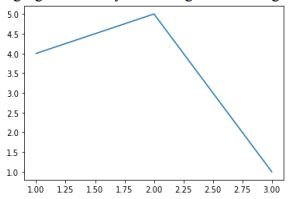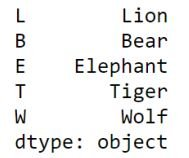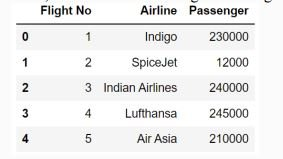# Term 1 Sample Paper Informatics Practices Class 12

## Term 1 Sample Paper Informatics Practices Class 12

Subject Code: 065

Time Allowed: 90 minutes

Maximum Marks: 35

General Instructions:
1. The paper is divided into 3 Sections- A, B and C.

2. Section A, consists of Question 1 to 25 and student need to attempt 20 questions.

3. Section B, consists of Question number 26 to 49 and student need to attempt 20 questions.

4. Section C, consists of Question number 50 to 55 and student need to attempt 5 questions.

5. All questions carry equal marks.

Section – A

Section A consists of 25 questions, attempt any 20 questions.

1. The name “Pandas” is derived from the term:

a) Panel Data

b) Panel Series

c) Python Document

d) Panel Data Frame

a) Panel Data

2. The command to install the pandas is:

a) install pip pandas

b) install pandas

c) pip pandas

d) pip install pandas

d) pip install pandas

3. Python pandas was developed by:

a) Guido van Rossum

b) Travis Oliphant

c) Wes McKinney

d) Brendan Eich

c) Wes McKinney

4. Pandas Series is:

a) 2 Dimensional

b) 3 Dimensional

c) 1 Dimensional

d) Multidimensional

c) 1 Dimensional

5. Pandas is a:

a) Package

b) Language

c) Library

d) Software

c) Library

6. We can analyse the data in pandas with

a) Series

b) Data Frame

c) Both of the above

d) None of the above

c) Both of the above

7. Out of the following, which function cannot be used for customization of charts in Python?

a) xlabel()

b) colour()

c) title()

d) xticks()

b) colour()

8. What is the minimum number of arguments required for plot() function in matplotlib?

a) 1

b) 2

c) 3

d) 4

a) 1

9. Method or function to add a new row in a data frame is:

a) .loc()

b) .iloc()

c) join

a) .loc()

10. _____________ is the function to save the graph.

a) Savefig()

b) Savefigure()

c) Savegraph()

d) Savechart()

a) Savefig()

11. A ______________ is a unique data trace of a user’s activities, actions, communications or transactions in digital media.

a) Digital Handprint

b) Digital Footprint

c) Offline Footprint

d) Offline Handprint

b) Digital Footprint

12. Which of the following is not a type of cybercrime?

a) Data theft

b) Forgery of online documents

c) Damage to data and systems

d) Installing antivirus for protection

d) Installing antivirus for protection

13. ‘F’ in FOSS stands for:

a) Free

b) Forever

c) Fire

d) Freezing

a) Free

14. Which of the following import statement is not correct?

a) import pandas as class12

b) import pandas as 1pd

c) import pandas as pd1

d) import pandas as pd

b) import pandas as 1pd

15. IPR stands for:

a) Intelligent Property Rights

b) Intellectual Property Reserve

c) Intellectual Property Rights

d) Intellectual Product Rights

c) Intellectual Property Rights

16. Which of the following is not a violation of IPR?

a) Plagiarism

c) Patent

c) Patent

17. While accessing the column from the data frame, we can specify the column name. In case column does not exist, which type of error it will raise:

a) Key Error

b) Syntax Error

c) Name Error

d) Runtime Error

a) Key Error

18. ____________is the practice of taking someone else’s work or ideas and passing them off as one’s own:

a) Plagiarism

c) Patent

d) All of the above

a) Plagiarism

19. Function to display the first n rows in the DataFrame:

a) tail (n)

c) top (n)

d) first (n)

20. Following are the impact of e-waste on the environment. Choose the odd one out.

a) Soil Pollution

b) Water Pollution

c) Air Pollution

d) Sound Pollution

d) Sound Pollution

21. Abdul deleted all his chats from all his social media accounts, and he thinks that all his traces are deleted completely. Is he right in thinking so?

a) Yes

b) No

c) May be

d) Not sure

b) No

22. The legal and regulatory aspects of the internet refer to…………………

a) Cyber Space

b) Cyber crime

c) Criminal law

d) IT act

d) IT act

23. Pandas data frame cannot be created using:

a) Dictionary of tuples

b) Series

c) Dictionary of List

d) List of Dictionaries

a) Dictionary of tuples

24. Which function will be used to read data from a CSV file into pandas data frame?

b) to_csv()

25. Which of the following is not an attribute of pandas data frame?

a) length

b) T

c) Size

d) shape

a) length

Section – B

Section B consists of 24 Questions (26 to 49). Attempt any 20 questions.

20. What will be the output of the given code?

```import pandas as pd

s = pd.Series([1,2,3,4,5], index=['akram','brijesh','charu','deepika','era'])

print(s['charu'])```

a) 1

b) 2

c) 3

d) 4

c) 3

27. Assuming the given series, named stud, which command will be used to print 5 as output?

Amit   90
Ramesh 100
Mahesh 50
john 67
Abdul 89
Name: Student, dtype: int64

a)  stud.index

b) stud.length

c) stud.values

d) stud.size

d) stud.size

28. A social science teacher wants to use a pandas series to teach about Indian historical monuments and its states. The series should have the monument names as values and state names as indexes which are stored in the given lists, as shown in the code. Choose the statement which will create the series:

```import pandas as pd

Monument=['Qutub Minar','Gateway of India','Red Fort','Taj Mahal']

a) S=df.Series(Monument,index=State)

b) S=pd.Series(State,Monument)

c) S=pd.Series(Monument,index=State)

d) S=pd.series(Monument,index=State)

c) S=pd.Series(Monument,index=State)

29. ____________ is a cybercrime in which a target or targets are contacted by email, telephone or text message by someone posing as a legitimate institution to lure individuals into providing sensitive data such as personally identifiable information, banking and credit card details, and passwords.

a) Plagiarism

b) Phishing

c) Cyberstalking

d) Hacking

b) Phishing

30. Observe the following figure. Identify the coding for obtaining this as output.```a) import matplotlib.pyplot as plt
plt.plot([1,2],[4,5])
plt.show()```

```b) import matplotlib.pyplot as plt
plt.plot([1,2,3],[4,5,1])
plt.show()```

```c) import matplotlib.pyplot as plt
plt.plot([2,3],[5,1])
plt.show()```

```d) import matplotlib.pyplot as plt
plt.plot([1,3],[4,1])
plt.show()```

```b) import matplotlib.pyplot as plt
plt.plot([1,2,3],[4,5,1])
plt.show()```

31. Read the statements given below and identify the right option to draw a histogram.

Statement A: To make a Histogram with Matplotlib, we can use the plt.hist() function.

Statement B: The bin parameter is compulsory to create histogram.

a) Statement A is correct

b) Statement B is correct

c) Statement A is correct, but Statement B is incorrect

d) Statement A is incorrect, but Statement B is correct

c) Statement A is correct, but Statement B is incorrect

32. Which graph should be used where each column represents a range of values, and the height of a column corresponds to how many values are in that range?

a) plot

b) line

c) bar

d) histogram

d) histogram

33. Suhhani is confused about the free operating system available in the market. Few of her friends suggested a few operating systems. Help her in choosing free operating system for her device:

a) Ubuntu

b) Windows

c) Mozilla Firefox

d) Blender

a) Ubuntu

34. Consider the following statements with reference to the use of email:

Statement 1: Sending threatening messages to a classmate

Statement 2: Inviting friends to participate in a school club or sports activity.

Statement 4: Responding to or forwarding spam emails.

Which of the above statements refer to appropriate use of email?

a) Statements 1 and 2.

b) Statements 2 and 3.

c) Statements 3 and 4.

d) Statements 2 and 4.

b) Statements 2 and 3

35. Consider the following series named animal:Write the output of the command:

print(animal[::-3])

a) L Lion

T Tiger

dtype: object

b) B Bear

E Elephant

dtype: object

c) W Wolf

B Bear

dtype: object

d) W Wolf

T Tiger

dtype: object

c) W Wolf

B Bear

dtype: object

36. Saharsh is a student of class -9 and he is a very frequent user of internet applications. One day he got an unpleasant message on his instant messenger. What do you think he should do?

a) ignore it and start chatting to someone else.

b) reply back to them and find out what their problem is.

c) shut down the computer and hope they’ll leave him alone in future.

d) go to his parents, teacher, or other trusted adult and let them know that he feels uncomfortable.

d) go to his parents, teacher, or other trusted adult and let them know that he feels uncomfortable.

37. What is a correct syntax to return the values of first row of a Pandas DataFrame? Assuming the name of the DataFrame is dfRent.

a) dfRent

b) dfRent.loc

c) dfRent.loc

d) dfRent.iloc

c) dfRent.loc

38. Feasible method (s) to manage e-waste:

a) Reduce

b) Reuse

c) Recycle

d) All of the above

d) All of the above

39. Difference between loc() and iloc().:

a) Both are Label indexed based functions.

b) Both are Integer position-based functions.

c) loc() is label based function and iloc() integer position based function.

d) loc() is integer position based function and iloc() index position based function.

c) loc() is label based function and iloc() integer position based function.

40. Write the output of the given program:

```import pandas as pd

S1=pd.Series([5,6,7,8,10],index=['v','w','x','y','z'])

l=[2,6,1,4,6]

S2=pd.Series(l,index=['z','y','a','w','v'])

print(S1-S2) ```

a)

a 0

v -1.0

w 2.0

x NaN

y 2.0

z 8.0

dtype: float64

b)

a NaN

v -1.0

w 2.0

x NaN

y 2.0

z 8.0

dtype: float64

c)

v -1.0

w 2.0

y 2.0

z 8.0

dtype: float64

d)

a NaN

v -1.0

w 2.0

x 3.0

y 2.0

z 8.0

dtype: float64

b)

a NaN

v -1.0

w 2.0

x NaN

y 2.0

z 8.0

dtype: float64

41. We should exhibit proper manners and etiquettes while being online. Choose the right net etiquette (s) from the following:

a) Avoid Cyber Bullying

b) Respect Other’s Privacy

d) All of the above

d) All of the above

42. Which command will be used to delete 3 and 5 rows of the data frame. Assuming the data frame name as DF.

a) DF.drop([2,4],axis=0)

b) DF.drop([2,4],axis=1)

c) DF.drop([3,5],axis=1)

d) DF.drop([3,5])

a) DF.drop([2,4],axis=0)

43. Write the output of the given command:

```import pandas as pd

s=pd.Series([1,2,3,4,5,6],index=['A','B','C','D','E','F'])

print(s[s%2==0])

```

a)

B 0

D 0

F 0

dtype: int64

b)

A 1

B 2

C 5

dtype: int64

c)

B 2

D 4

F 6

dtype: int64

d)

B 1

D 2

F 3

dtype: int64

c)

B 2

D 4

F 6

dtype: int64

44. Ritika is a new learner for the python pandas, and she is aware of some concepts of python. She has created some lists, but is unable to create the data frame from the same. Help her by identifying the statement which will create the data frame.

```
import pandas as pd

Name=['Manpreet','Kavil','Manu','Ria']

Phy=[70,60,76,89]

Chem=[30,70,50,65] ```

a) df=pd.DataFrame({“Name”:Name,”Phy”:Phy,”Chem”:Chem})

b) d=(“Name”:Name,”Phy”:Phy,”Chem”:Chem) df=pd.DataFrame(d)

c) df=pd.DataFrame([Name,Phy,Chem],columns=[‘Name’,”Phy”,”Chem”,”Total”])

d) df=pd.DataFrame({Name:”Name”, Phy :”Phy”,Chem: “Chem”})

a) df=pd.DataFrame({“Name”:Name,”Phy”:Phy,”Chem”:Chem})

45. Linux, MySQL and Mozilla Firefox software come under_______ category.

a) Proprietary

b) FOSS

c) Freeware

d) Shareware

b) FOSS

46. Assuming the given structure, which command will give us the given output:Output Required: (3,5)

a) print(df.shape())

b) print(df.shape)

c) print(df.size)

d) print(df.size())

b) print(df.shape)

47. Write the output of the given command: df1.loc[:0,’Sal’] Consider the given dataframe.

a)  0 Kavita 50000 3000

b)  50000

c)  3000

d)  50000

b)  50000

48. Consider the following data frame name df Write the output of the given command:

print(df.marks/2)

a)

0 45.0

1 NaN

2 43.5

Name: Marks, dtype: float64

b)

0 45.0

1 NaN

2 43

Name: Marks, dtype: float64

c)

0 45

1 NaN

2 43.5

Name: Marks, dtype: float64

d)

0 45.0

1 0

2 43.5

Name: Marks, dtype: float64

a)

0 45.0

1 NaN

2 43.5

Name: Marks, dtype: float64

49. Read the statements given below. Identify the right option from the following for Attribute and method/function.

Statement A: Attribute always ends without parenthesis.

Statement B: Function/Method cannot work without arguments.

a) Both statements are correct.

b) Both statements are incorrect.

c) Statement A is correct, but Statement B is incorrect

d)  Statement A is incorrect, but Statement B is correct

c) Statement A is correct, but Statement B is incorrect

Section – C
Section C, consists of 6 Question (50 to 55). Attempt any 5 questions.

## Case Study

Mr. Sharma is working with an IT company, and he has provided some data. On which he wants to do some operations, but he is facing some problem, help him:

### Code:

import pandas as pd

ResultSheet={

‘Naveen’: pd.Series([90, 91, 97],

index=[‘Maths’,’Science’,’Hindi’]),

‘Rehana’: pd.Series([92, 81, 96],

index=[‘Maths’,’Science’,’Hindi’]),
‘John’: pd.Series([89, 91, 88],

index=[‘Maths’,’Science’,’Hindi’]),

‘Roja’: pd.Series([81, 71, 67],

index=[‘Maths’,’Science’,’Hindi’]),

‘Mannat’: pd.Series([94, 95, 99],

index=[‘Maths’,’Science’,’Hindi’])}

DF = pd.DataFrame(ResultSheet)

print(DF)

### Output of the above code:

Based on the given information, answer the questions NO. 50-55.

50. He wants to add a new column with name of student ‘Prem’ in above data frame choose the right command to do so:

a) DF[‘Prem’]=[89,78,76]

b) df[‘Prem’]=[89,78,76]

c)  DF[‘Prem’]=[89,78,76,67]

d) DF[‘Name’]=[89,78,76]

a) DF[‘Prem’]=[89,78,76]

51. He wants to set all the values to zero in data frame, choose the right command to do so:

a) DF=0

b) DF[]=0

c) DF[:]=0

d) DF[:]==0

c) DF[:]=0

52.He wants to delete the row of science marks:

a)  DF.drop(‘Science’, axis=1)

b) DF.drop(‘Science’, axis=0)

c) DF.drop(‘Science’, axis=-1)

d) DF.drop(‘Science’, axis==0)

b) DF.drop(‘Science’, axis=0)

53. The following code is to create another data frame, which he wants to add to the existing Data frame. Choose the right command to do so:

Sheet1={
index=[‘Maths’,’Science’,’Hindi’])}
S1=pd.DataFrame(Sheet1)

a) DF.append(S1,axis=0)

b) DF.append(S1)

c) DF.insert(S1)

d) DF.join(S1)

b) DF.append(S1)

54. What will be the output of the given command?
DF.index=[‘A’,’B’,’C’]

a)
Naveen
Rehana
John
Roja
Mannat
A
Maths
90
92
89
81
94
B
Science
91
81
91
71
95
C
Hindi
97
96
88
67
99

b.
c.
d. Error, Index already exists and cannot be overwritten.

b)

55. What will be the output of the given command?
print(DF.size)

a) 15

b) 18

c) 21

d) 23

Naveen
Rehana
John
Roja
Mannat
A
90
92
89
81
94
B
91
81
91
71
95
C
97
96
88
67
99
A
B
C
Roja
Mannat
Maths
90
92
89
81
94
Science
91
81
91
71
95
Hindi
97
96
88
67
99

a) 15#### By cbsepython

A complete solution for the students of class 9 to 12 having subject Information Technology (402), Computer Science (083). Explore our website for all the useful content as Topic wise notes, Solved QNA, MCQs, Projects and Quiz related to the latest syllabus.